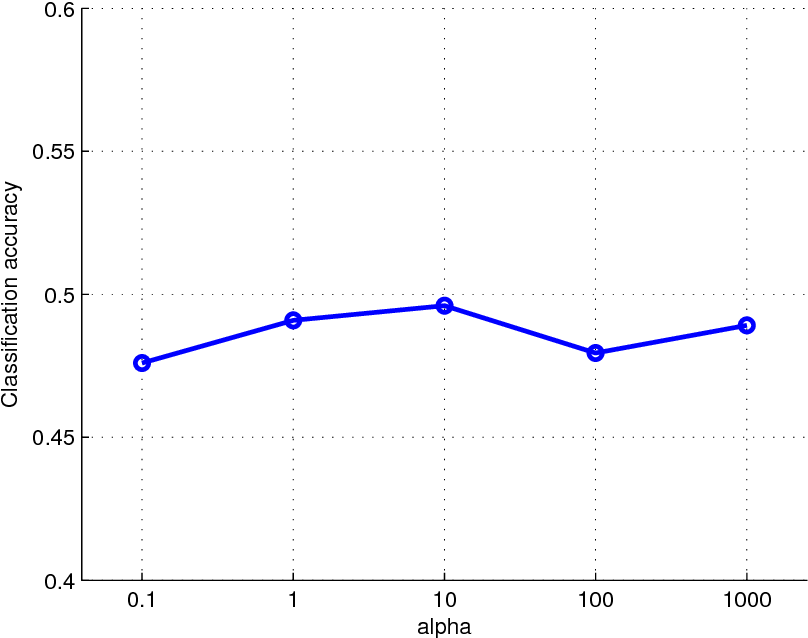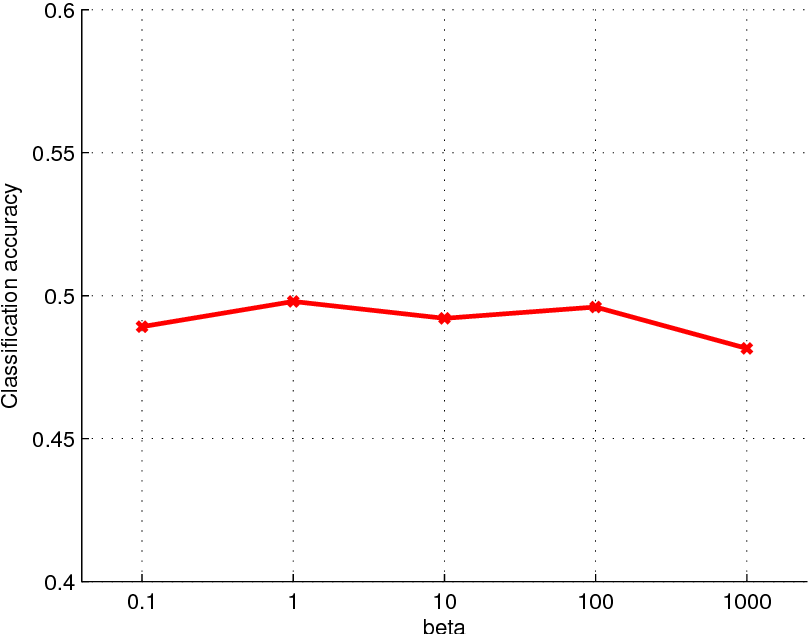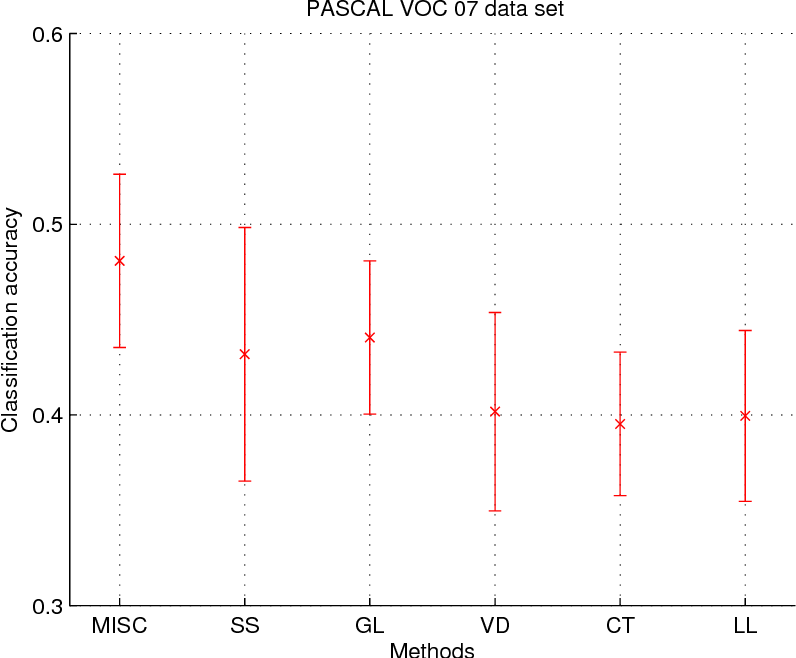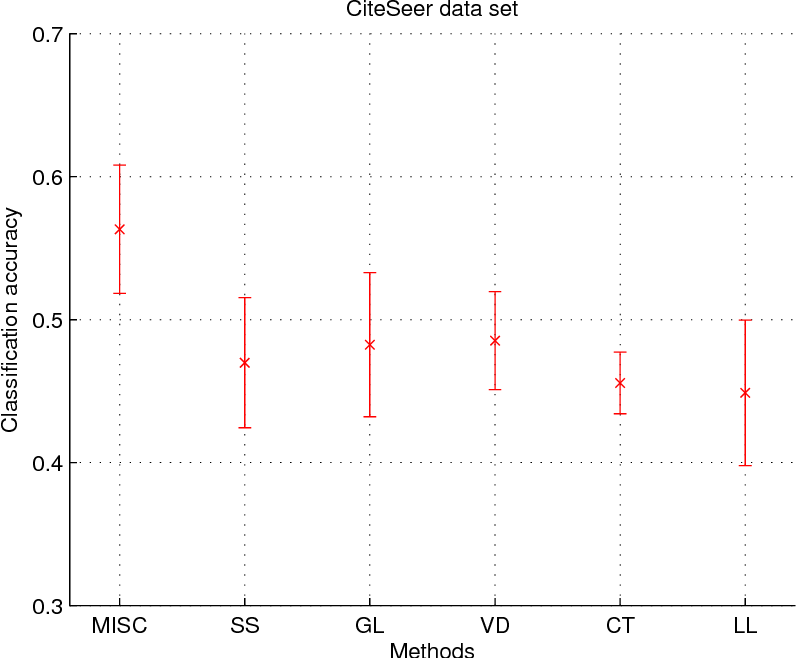Research papers and code for "Qingjun Wang":Multiview learning problem refers to the problem of learning a classifier from multiple view data. In this data set, each data points is presented by multiple different views. In this paper, we propose a novel method for this problem. This method is based on two assumptions. The first assumption is that each data point has an intact feature vector, and each view is obtained by a linear transformation from the intact vector. The second assumption is that the intact vectors are discriminative, and in the intact space, we have a linear classifier to separate the positive class from the negative class. We define an intact vector for each data point, and a view-conditional transformation matrix for each view, and propose to reconstruct the multiple view feature vectors by the product of the corresponding intact vectors and transformation matrices. Moreover, we also propose a linear classifier in the intact space, and learn it jointly with the intact vectors. The learning problem is modeled by a minimization problem, and the objective function is composed of a Cauchy error estimator-based view-conditional reconstruction term over all data points and views, and a classification error term measured by hinge loss over all the intact vectors of all the data points. Some regularization terms are also imposed to different variables in the objective function. The minimization problem is solve by an iterative algorithm using alternate optimization strategy and gradient descent algorithm. The proposed algorithm shows it advantage in the compression to other multiview learning algorithms on benchmark data sets.

Click to Read Paper and Get Code